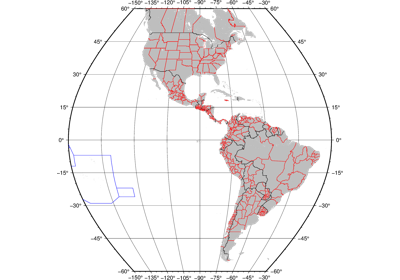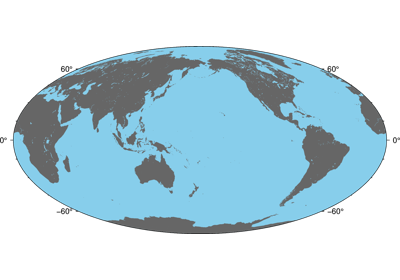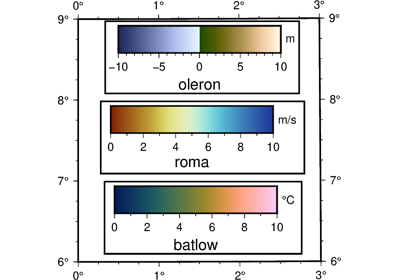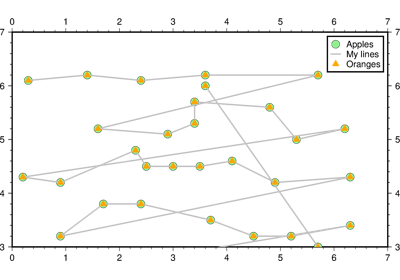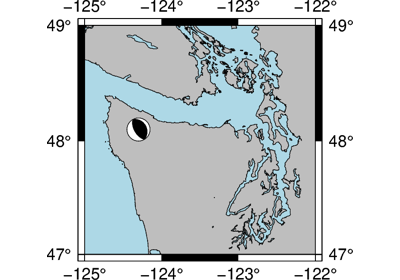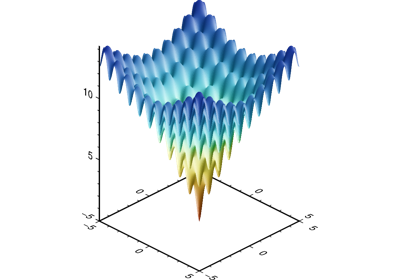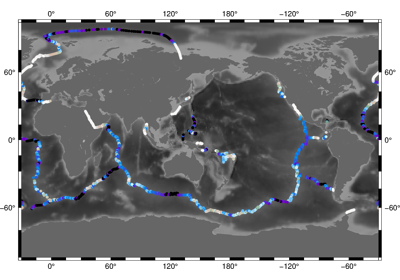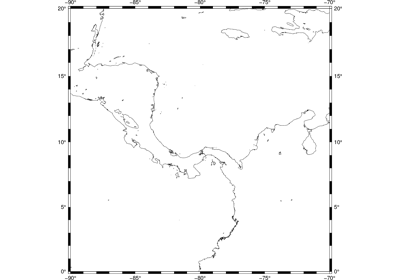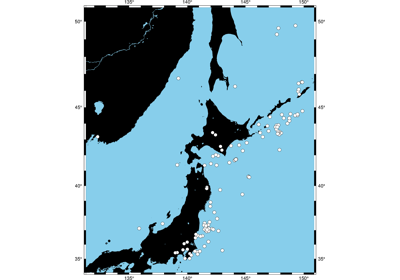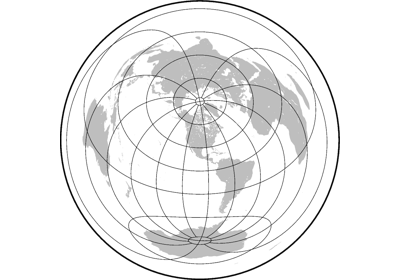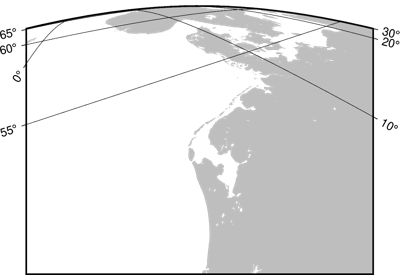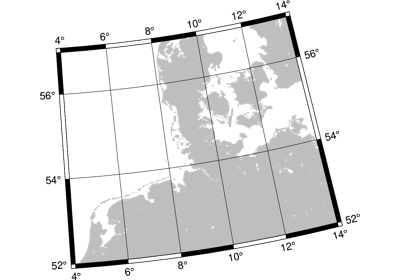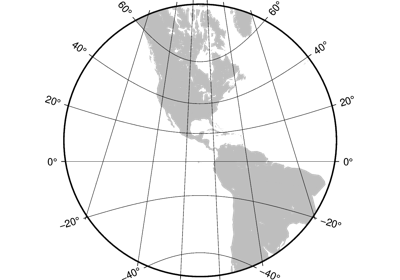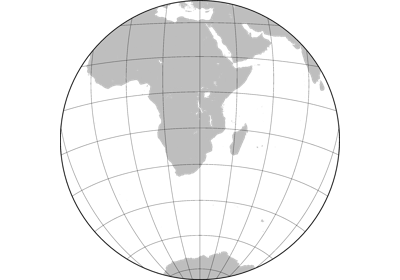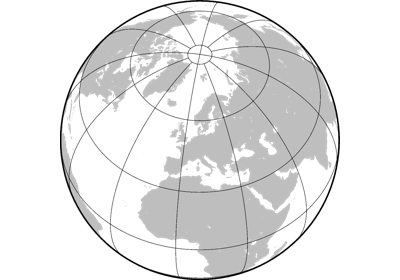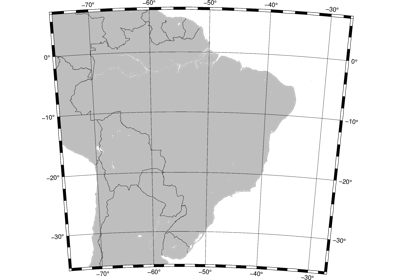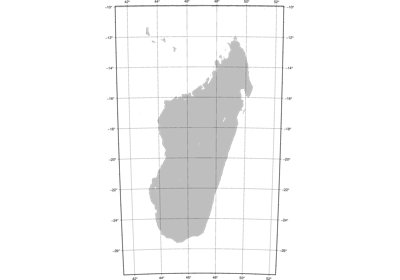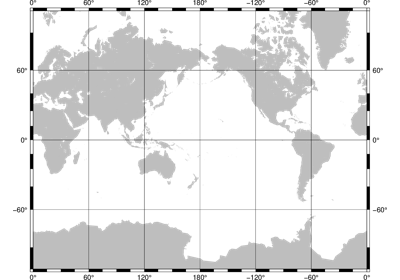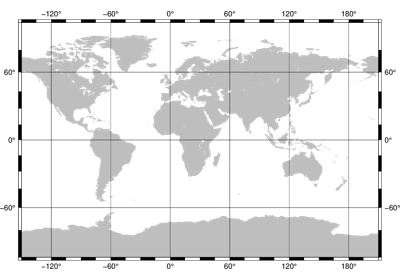# pygmt.Figure¶

class pygmt.Figure[source]

A GMT figure to handle all plotting.

Use the plotting methods of this class to add elements to the figure. You can preview the figure using pygmt.Figure.show and save the figure to a file using pygmt.Figure.savefig.

Unlike traditional GMT figures, no figure file is generated until you call pygmt.Figure.savefig or pygmt.Figure.psconvert.

Examples

>>> fig = Figure()
>>> fig.basemap(region=[0, 360, -90, 90], projection='W7i', frame=True)
>>> fig.savefig("my-figure.png")
>>> # Make sure the figure file is generated and clean it up
>>> import os
>>> os.path.exists('my-figure.png')
True
>>> os.remove('my-figure.png')


The plot region can be specified through ISO country codes (for example, 'JP' for Japan):

>>> fig = Figure()
>>> fig.basemap(region='JP', projection="M3i", frame=True)
>>> # The fig.region attribute shows the WESN bounding box for the figure
>>> print(', '.join('{:.2f}'.format(i)  for i in fig.region))
122.94, 145.82, 20.53, 45.52


Methods Summary

 Figure.basemap(**kwargs) Produce a basemap for the figure. Figure.coast(**kwargs) Plot continents, shorelines, rivers, and borders on maps Figure.colorbar(**kwargs) Plot a gray or color scale-bar on maps. Figure.contour([x, y, z, data]) Contour table data by direct triangulation. Figure.grdcontour(grid, **kwargs) Convert grids or images to contours and plot them on maps Figure.grdimage(grid, **kwargs) Project grids or images and plot them on maps. Figure.grdview(grid, **kwargs) Create 3-D perspective image or surface mesh from a grid. Figure.image(imagefile, **kwargs) Place images or EPS files on maps. Figure.legend([spec, position, box]) Plot legends on maps. Figure.logo(**kwargs) Place the GMT graphics logo on a map. Figure.meca(spec, scale[, longitude, …]) Plot focal mechanisms. Figure.plot([x, y, data, sizes, direction]) Plot lines, polygons, and symbols on maps. Figure.psconvert(**kwargs) Convert [E]PS file(s) to other formats. Figure.savefig(fname[, transparent, crop, …]) Save the figure to a file. Figure.shift_origin([xshift, yshift]) Shift plot origin in x and/or y directions. Figure.show([dpi, width, method]) Display a preview of the figure. Figure.text([textfiles, x, y, position, …]) Plot or typeset text strings of variable size, font type, and orientation.

## Examples using pygmt.Figure¶